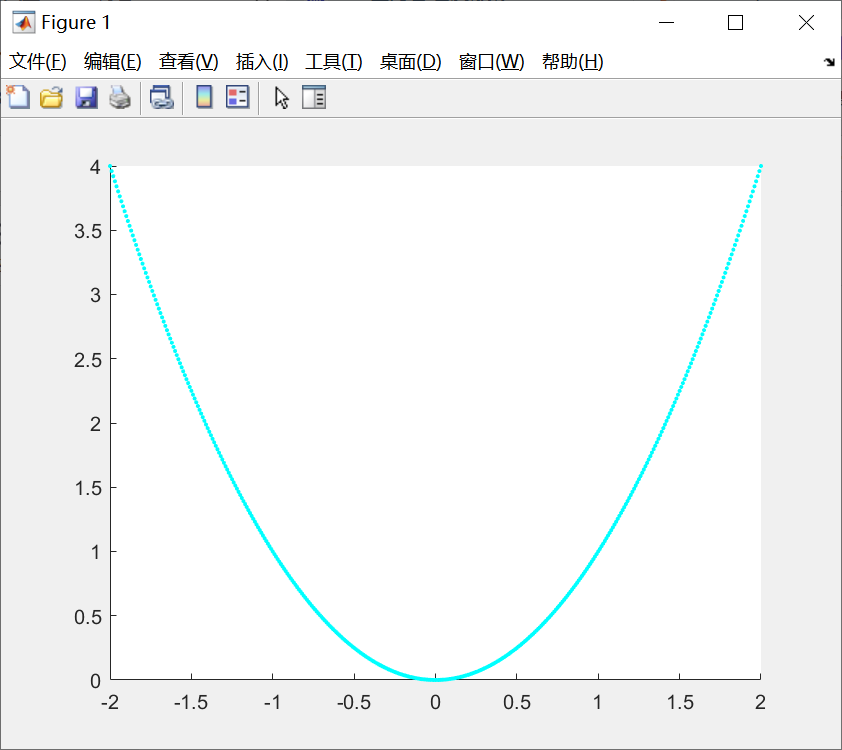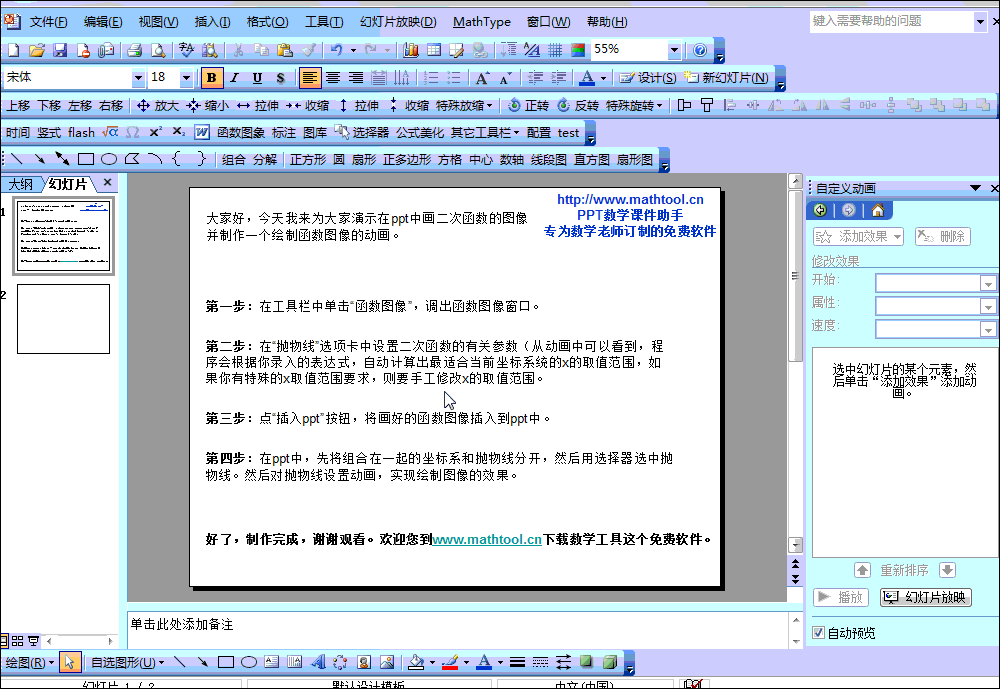• 是在学习了一次函数的基础上再进行研究的，在学习时要学会掌握画函数图像的方法，之前都是借助直尺来画函数图像的，现在可以借助专业的绘图工具来完成，下面就一起来学习快速画二次函数图像的方法。 绘图工具几何...

二次函数是中学时代必学的一类基本初等函数，是在学习了一次函数的基础上再进行研究的，在学习时要学会掌握画函数图像的方法，之前都是借助直尺来画函数图像的，现在可以借助专业的绘图工具来完成，下面就一起来学习快速画二次函数图像的方法。
绘图工具几何画板免费获取地址：http://wm.makeding.com/iclk/?zoneid=13398
以f(x)=2x2+3x-5为例，具体操作步骤如下：
一、绘制函数：
1.在“绘图”菜单中选择“绘制新函数”命令，出现“新建函数”对话框。在“绘图”菜单中选择“绘制新函数”的命令

2.输入函数表达式。在“新建函数”对话框中，按对话框上的数字按钮输入函数图像点击“确定”，自动生成f(x)=2x2+3x-5的函数图像。新建函数对话框中输入函数表达式

3. f(x)=2x2+3x-5的图像如图所示。二次函数f(x)=2x
2+3x-5的图像示例

二、调整图像：
1.整体移动。单击“移动箭头工具”，在坐标系中按住坐标原点拖动可以移动整个坐标系的位置。选中坐标原点整体移动函数图像

2.调整数据。如果觉得数据不够精确，或者太详细了，你可以单击“移动箭头工具”，然后在选中X轴上的红点并拖动可以放大或者缩小刻度。选中X轴与函数图象的交点拖动调节刻度值

以上就是给大家介绍的快速画二次函数图像的方法，不管是新手还是老用户，都可以很快地掌握这种简单的几何绘图方法。除此之外，还可以画任意普通函数，具体教程可访问：www.jihehuaban.com.cn/xinshourumen/hanshu-tuxiang.html
以上文章转载自：http://www.jihehuaban.com.cn/xinshourumen/huizhi-hanshu.html

展开全文• 二次函数图像生成器是一款可以根据二次函数出相应的抛物线的工具，二次函数图像生成器支持多条二次函数在同一坐标轴上。能显示与坐标轴的交点，并且有动画等功能，是工程、解题的有力帮手。    更新日志 ： ...
• 本软件可以根据您的二次函数出相应的图象，支持多条二次函数画在同一坐标轴上。能显示与坐标轴的交点，并且有动画等功能，是工程、解题的有力帮手。
• 本软件可以根据您的二次函数出相应的抛物线，支持多条二次函数画在同一坐标轴上。能显示与坐标轴的交点，并且有动画等功能，是工程、解题的有力帮手。 修复了抛物线消失的bug，支持清除抛物线等。
• 2.3.2例子二次函数绘制方法把二次函数图像变成点存储于list中直线的时候我们了三个点，我们还可以更多的点。点越多图像就越是一条直线，运用的思想是微积分的思想。如何生成很多点呢?python本身自带range()...
2.3.1工具
python
matplotlib
pyplot
pyplot四Matplotlib中的模块，封装了很多处理graph的方法。绘制表格，修改表格属性都是用它实现的。
2.3.2例子
二次函数绘制方法
把二次函数图像变成点存储于list中
画直线的时候我们画了三个点，我们还可以画更多的点。点越多图像就越是一条直线，运用的思想是微积分的思想。如何生成很多点呢?python本身自带range()函数， 我们可以使用它来自动生成点的横坐标，但是range()只能自动生成间隔为自然数的数据。我们就需要自己写一个函数自动生成任意间隔的数据。
def frange(start,final,increment):
numbers=[]
while start
numbers.append(start)
start = start + increment
return numbers
a= frange(1,5,0.2)
print a
frange()函数是我们自己写的，用来生成任意间隔的数据。
在图表中显示出这些点。
from pylab import plot,show
这个方法是引用pylab模块，导入pylab模块的方法，然后就可以直接使用这些方法。
import matplotlib.pyplot as plt
这个方法是导入模块，然后实例化一个pyplot类对象。然后通过对象直接使用pyplot模块的方法，和上面的方法效果一样。
plt.style.use('ggplot')#使用"ggplot风格美化图表’
def create_graph(a,b,c,d,e):#定义图表函数
x = frange(d,e,0.02)#生成x轴坐标
y = []#定义y轴坐标数据类型
for i in x:
y.append(a*i**2+b*i+c)#y轴坐标数据计算
plt.plot(x,y)#把x、y轴坐标带入plot（）画图。
plt.xlabel(u'x轴')#显示x轴名词，u是更改字符编码。
plt.ylabel(u'y')
plt.title(u'二次函数图像')
plt.show()#展示出来。
create_graph(1,2,1,-4,3)
如果直接在Matplotlib中使用中文会出现
报错：ValueError: matplotlib display text must have all code points < 128 or use Unicode strings
中文编码问题的解决方法只需在中文字符串前面加个u即可，表示使用Unicode编码。2.3.3有没有现成的生成x轴坐标的python库
当然有现成的生成x轴坐标的库，numpy就是其中一个。NumPy是Python中科学计算的基础软件包。它是一个提供多了维数组对象，多种派生对象（如：掩码数组、矩阵）以及用于快速操作数组的函数及API，它包括数学、逻辑、数组形状变换、排序、选择、I/O 、离散傅立叶变换、基本线性代数、基本统计运算、随机模拟等等。
使用方法
import numpy as np
x = np.arange(-5, 5, 0.01)#可以自动生成x轴坐标，最小间隔为0.01
上面程序可以修改为
#-*- coding:utf-8 -*-
import matplotlib.pyplot as plt
import numpy as np
plt.style.use('ggplot')#使用"ggplot风格美化图表’
def create_graph(a,b,c,d,e):
x = np.arange(d,e,0.01)
y = a*x**2+b*x+c
plt.plot(x,y)
plt.xlabel(u"x轴")
plt.title(u'二次函数')
plt.show()
create_graph(1,2,3,-4,4)
这次函数写的简单多了，而且y值也不需要用for循环来生成，直接使用即可。
2.3.4 绘制数学函数图像
numpy功能很强大，可以涵盖很多数学函数，直接拿来使用即可。
import matplotlib.pyplot as plt
import numpy as np
pai =np.pi
x = np.arange(0,2*pai,0.01)
y = np.sin(x)
plt.plot(x,y)
plt.show()
例如正弦函数图像感想
以前自己总是纳闷，计算上的函数图像到底是如何生成的，而且纳闷细腻，经过深入了解函数图像的生成过程，才懂得函数图像是一个点一个点绘制出来的，只要x轴数据足够多，加上计算机的强大计算能力，很快就能绘制出函数图像来。事情并不难，难的是没有方式和方法。
展开全文• 二次函数图像 clc,clear; hold on for x=-2:0.01:2 y=x^2; plot(x,y,'c.'); end
二次函数图像
clc,clear;
hold on
for x=-2:0.01:2
y=x^2;
plot(x,y,'c.');
end展开全文matlab
•展开全文动画教程
• 在学习二次函数的时候，我们学习过用描点法来大概画出二次函数，在几何画板中我们可以也用描点法准确的画出二次函数，下面将详细介绍几何画板如何用描点法画二次函数。 具体的操作步骤如下： 第一步 定义三个坐标点 ...python
• # mpl_toolkits是matplotlib官方的工具包 mplot3d是用来三维图像的工具包 from mpl_toolkits.mplot3d import Axes3D # pyplot 是一个有命令风格的的函数集合，与matlab相似。 from matplotlib import pyplot as ...
• 一个用swing写的一个画二次函数图象的工具,有源代码。适合初学着
• 这是一个二次函数图像的flash课件，请下载使用。flash课件
• 包含了源码，有函数的设置，还有exe可执行的文件
• 基于Matlab的图形用户界面（GUI）设计，该算法实现了一元二次函数y=ax^2+bx+c的图形绘制，其中a、b、c为可输入参数，能够多次绘制函数图像，也能叠加绘制函数图像
• 基于Matlab的图形用户界面（GUI）设计，该算法实现了一元二次函数y=ax^2+bx+c的图形绘制，其中a、b、c为可输入参数，能够多次绘制函数图像，也能叠加绘制函数图像
• matplotlib 基础练习:一元二次函数 样例： 1、安装matplotlib模块 Matplotlib 是 Python 的绘图库。 安装方法： pip install matplotlib -i ...python numpy matlab
• 原理。。 将每个像素对应的x,y值带入，算出Ax^2+Bxy+Cy^2+Dx+Ey+F 若大于零，数组中该点值为1，否则为0 然后、。。。。。...界面极其粗制滥造，“用”表示使用这个参数，不选则该常数为0，“负”表示设置该项为负数...
• 二次函数是中学时代必须要学习的一类基本...几何画板作为好用的绘图工具，可以快速二次函数图像，该软件免费获取地址：http://wm.makeding.com/iclk/?zoneid=13398 二次函数图象的变换包含上下左右平移、轴对称...
• 废话不多说，上图。 f(x)=x² ...代码与一次函数基本没什么变化，这里加了个a文本框的分数转换，由于二次函数为曲线，那么最直接的办法就是用多个点然后连接起来。 #region 二次函数 private vo线性代数
• 今天是高考第二天了，看了一会昨天高考数学卷，刚好又在学习canvas这部分内容，所以突发奇想的或一个二次曲线图。首先，我们需要创建一个400*800的画布并让其居中显示&lt;canvas id="drawing" width=&...canvas canvas应用
• 在二次函数学习中，不同形式二次函数图像的平移变换与函数解析式之间的关系可以通过几何画板直观清晰地进行表达，下面就一起来学习具体的制作技巧。 最新版几何画板软件获取地址：http://wm.makeding.com/ic...
• 我们都知道，一个定义在R上的二次函数，对应一条抛物线，R2R^2R2上对应图形有以下几种： 一件有趣的事情是，线性代数 高等代数 对称矩阵
• X, Y = np.meshgrid(x, y) # 网格的创建，生成维数组 #X=np.array(x) #Y=np.array(y) print(type(X),X) #Z = np.sin(X) * np.cos(Y) #Z=X 4+Y 2 #Z=-X 2-Y 2 #Z=2 X+2 Y Z=4*np.sin(X)+Y**2 #print(type...
• 用VB6写的函数图象方法 主要是二次函数；正弦余弦等
• 今天小编就为大家分享一篇使用python绘制二元函数图像的实例，具有很好的参考价值，希望对大家有所帮助。一起跟随小编过来看看吧
• 第22章二次函数全章复习教案【学习目标】　1．通过对实际问题情境的分析确定二次函数的表达式，并体会二次函数的意义；...会用描点法二次函数图象，能从图象上认识二次函数的性质；　3．会根据公式确定
• sigmoid激活函数： import numpy as np import matplotlib.pylab as plt def sigmoid(x): return 1/(1+np.exp(-x)) # sigmoid函数 x = np.arange(-6.0,6.0,0.1) # 限定x的范围，给什么区间出来的就是在哪个...python 开发语言 后端
•数学知识 优化问题
• 看到可以绘制函数图像，便简单的试验了一下。实现结果如下所示：   实现代码如下：     X2Function.java import javax.swing.*; import java.awt.*; public class X2Function extends JFrame { ...编程 Java Swing...